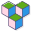CppBuzz# Java program to add two integer number

``````public class Main {
public static void main(String[] args) {

int a = 5;
int b = 5;
int sum = a + b;

System.out.println("Addition of 2 integer is : " + sum);

// there are many ways we can add 2 integer

System.out.println(5+5);

System.out.println(a+b);
}
}
``````